How to Learn in 24 Hours?The Rapid Learning Movie

 Need Help? M-F: 9am-5pm(PST): Toll-Free: (877) RAPID-10 US Direct: (714) 692-2900 Int'l: 001-714-692-2900 24/7 Online Technical Support: The Rapid Support Center Secure Online Order:Got Questions? Frequently Asked Questions
 Need Proof? Testimonials by Our Users
 Trustlink is a Better Business Bureau Program. Rapid Learning Center is a fivr-star business. External TrustLink Reviews

 Rapid Learning Courses: MCAT in 24 Hours (2015-16) USMLE in 24 Hours (Boards) Chemistry in 24 Hours Biology in 24 Hours Physics in 24 Hours Mathematics in 24 Hours Psychology in 24 Hours SAT in 24 Hours ACT in 24 Hours AP in 24 Hours CLEP in 24 Hours DAT in 24 Hours (Dental) OAT in 24 Hours (Optometry) PCAT in 24 Hours (Pharmacy) Nursing Entrance Exams Certification in 24 Hours eBook - Survival Kits Audiobooks (MP3)

 Tell-A-Friend: Have friends taking science and math courses too? Tell them about our rapid learning system.Home »  Mathematics »  Pre-Calculus

Limits and Rates of Change

 Topic Review on "Title": Definition of limits: Let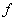be a function,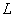is the limit of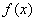at the point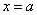if and only if for any, there is a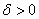such that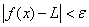whenever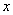satisfies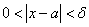. Limit operations: The sum of the limits of two functions is equal to the sum of the first limit plus the sum of the second limit. The product of the limits of two functions is equal to the first limit times the second limit. The quotient of the limits of two functions is equal to first limit divided by the second limit.  Definition of continuity of functions: Letbe a function,is said to be continuous atif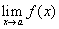exists and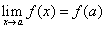.   Derivative Operations: Let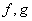be functions. Ifand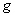are both differentiable atthen 1)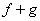is differentiable atand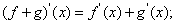2)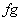is differentiable atand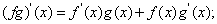3) Ifthenis differentiable atand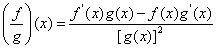.

Rapid Study Kit for "Title":
 Flash Movie Flash Game Flash Card Core Concept Tutorial Problem Solving Drill Review Cheat Sheet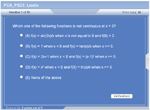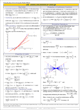"Title" Tutorial Summary : This tutorial discusses the limits and the rates of change. Knowing the concept of limit process and instantaneous change is important to the formulation of derivatives and approximation of solutions. The different types of limits that one gets are discussed in the graphical illustrations. Properties of limits and limit operations are presented so an evaluation of limits can be performed. An example of how a function can have no limit but be bounded by 1 is discussed. The principle of continuity relies on limits and it has properties that are useful with the rates of change concept.

 Tutorial Features: Specific Tutorial Features: • Graphical illustration of one sided limits and the continuity of functions is shown in the examples. • Typical rate of change problems are introduced with graphical interpretations. Series Features: • Concept map showing inter-connections of new concepts in this tutorial and those previously introduced. • Definition slides introduce terms as they are needed. • Visual representation of concepts • Animated examples—worked out step by step • A concise summary is given at the conclusion of the tutorial.

 "Title" Topic List: Limit of functions Definition of limits Limit operations Properties of limits One sided limitsContinuity Definition of the continuity of functions One sided continuity Properties of continuous functionsRate of change Derivative operations

See all 24 lessons in Pre-Calculus, including concept tutorials, problem drills and cheat sheets:
Teach Yourself Pre-Calculus Visually in 24 Hours